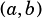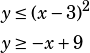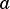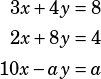1If the system of inequalities above is graphed in the-plane and the ordered pairis in the solution set, then which of the following statements cannot be disproven?

A)
B)
C)
D)
2

Which of the following statements CANNOT be true about a set of 10 numbers?

1. The median of the 10 numbers is one of the 10 numbers
2. The sum of the 10 numbers is equal to the average (arithmetic mean) of the 10 numbers
3. The set of 10 numbers has 6 modes.
A)
B)
C)
D)
3In the-plane, a point with coordinateslies in the solution set of the system of inequalities above. Ifandare greater than zero, what is the least possible value of?

4If the three equations above are graphed in the-plane, they form three lines that intersect at one point. What is the value of the constant?

5In the system of equations above,is a constant. When the equations are graphed in the-plane, they form parallel lines. What is the positive difference between the-intercepts of the two lines?
A)
B)
C)
D)

Section progress